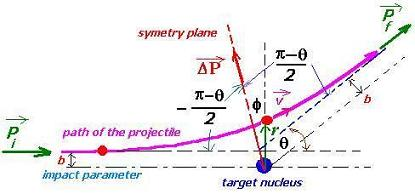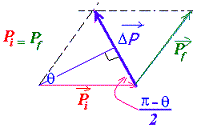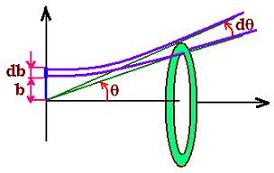Projectile's charge Z Projectile's E (MeV)

Contents

The nucleus

Statistical Mechanics

Related topics

# Rutherford scattering

### 1. The description of the collision

The main idea, here is the discovery of the proton. Geiger and Marson experimented the collision of α particles (of energy 5.5 Mev) from the Radon Rn(222,86); that is the nuclei of the He(4,2) incident on the gold Au(197,79) target (of width 10-6m = 1 µm).
Geiger and Marson found about 1 on 104 α are returned back (scattered in the direction θ>π/2).

J.J. Thomson had thought; that is the the atom was like a pulm ( or raisin) pudding, where the pudding was positive charges and raisin electrons. This is the first atomic model that it doesn't work at all.

With the rutherford experiment, It came out that the incident particles had a small amount of deflections and even some particles returned from the target.
The conclusion was that the atom is made up of the positive nucleus and electrons where the biggest part is simply a vacuum. This second model gave a good understanding about the atom.

Features of the collision:
Projectile:
m : Mass of the projectile
vi : Initial speed of the projectile
vf : Final speed of the projectile
Z1e : Charge : (Z : atomic number, e : electron charge)
Pi : Momentum
Ni : Number of incident (alpha) particles
T = (1/2)mvii2 : kinetic energy of projectile ( a )
Pi = mvi : initial momentum
Li = mvib : initial angular momentum
Lf = mvfb : initial angular momentum
Eα = E i = (1/2) mvi2 : The kinetic energy of the incident particle
Eα = (1/2)mvi2
Target nuclei:
M : Mass of the target
Z2e : Charge
Pf : Final momentumDetection parameters:
ε0 : Permittivity of space
θ: Scattering angle
φ : The angle between the vector position and ω = dφ/dt : The angular velocity of the particle at any moment the axis of the hyperbola
r : Position vector; the radial unit vector (||r|| = 1).
d : Target-to-detector distance
L = mvr = mωr2 : Angular momentum at any moment
b : Impact parameterThe scattering under the influence of the Coulomb force involve the dependence of the scattering θ angle and the impact parameter b which is the crucial parameter for nuclear scattering.

We Consider the M>>m. So,the magnitudes of the initial and final momenta are the same considering their conservation and the assumption that the target recoil is negligible:

Let:
ΔP = ||ΔP|| = ||Pf - Pi|| = 2 mvi sin(θ/2) (1)
The Coulomb vector force is expressed by:
F = (1/4πε0) Z1Z2e2 r/r2 (2)
r : is the position of the projectile from the target at any moment.

In view of the symmetry of the scattering geometry, the net change of this force is located in the symmetry axis of the hyperbola. Therefore:
FΔP = F cos φ (3)

Recall that F = dP/dt; hence : P = ∫ F dt.
And L:the angular momentum is conserved; that is Li = Lf = L = mvr = mωr2;
Li = L gives: mvib = mωr2
Hence:
r2/vib = 1/ω = dt/dφ (4)

We have then:
ΔP = ∫FΔP dt ( from -&infinit to +&infinit)
= ∫F cos φ dt ( from : - (π-θ)/2 to + (π-θ)/2)
= ∫dφF cos φ (dt/dφ)
= ∫dφF cos φ(r2/vib)
= (Z1Z2e2/4πε0vib) &int:cos φdφ ( from : - (π -θ)/2 to + (π-θ)/2)
= (Z1Z2e2/4πε0vib) . 2 cos(θ/2) (5)

Equating the relationships (1) and (5) we get:
b = (Z1Z2e2/4πε0mvi2) ctg(θ/2)
Since Eα = (1/2) mvi2, we have then:
b = (1/2)(Z1Z2e2/4πε0 Eα) ctg(θ/2) (6)

Let's write :
rc = (Z1Z2e2/4πε0) x 1/ Eα
That is obtained with φ = 0 and b = 0
In this case, the kinetic enrgy of the incident particle is null; wheras the potential energy takes the expression:
Ep = Z1Z2e2/4πε0rc

Recall that the size of the nucleus is given by the formula :
rnucleus = 1.2 A 1/3. Where A is the mass of the nucleus
For the gold element A = 197, thus rnucleus = 8 fm
For the current Rutherford collision : rc ≈ 30 fm
and the Bohr radius ≈0.5 105 fm

The expression of the impact parameter takes then the form:

b = (rc/2) ctg (θ/2) (7)

wich is the Rutherford formula.

### 2. The related cross sectionThe differential cross section gives information about the probability for the insident α to be scattered in a certain direction.
The total cross section is expressed by:
σ = π b2    (8)
Then:
dσ = 2π b db
dΩ = 2πsinθdθ
dσ = 2π b db sinθdθ / sinθdθ = dΩ b db / sinθdθ
Where Ω is the related solid angle.
Hence;
dσ/ dΩ = (b / sinθ)(db /dθ)    (9)
From the equation (7), we get:
|db / dθ| = rc/2 . (1/2 sin2(θ/2)    (10)

The equation (9) becomes:
dσ/ dΩ = (rc/2 ctg(θ/2)/sinθ) / rc/2 . (1/2 sin2(θ/2)
(11) With sin θ = 2 sin(θ/2) cos&(θ/2), we find:

dσ/dΩ = (rc/4)2 1/sin4(θ/2)Web ScientificSentence